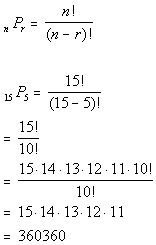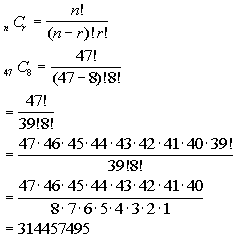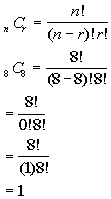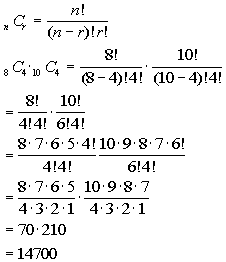TitleCollege Algebra
Tutorial 57: CombinationsAnswer/Discussion to 1a The order of the demonstrators is important?

Keeping in mind that order is important, would this be a permutation or a combination?

If you said a permutation problem, give yourself a pat on the back..

First we need to find n and r :

 If n is the number of students we have to choose from,  what do you think n is in this problem? If you said n = 15 you are correct!!!  There are 15 students. If r is the number of students chosen at a time, what do you think r is? If you said r = 5, pat yourself on the back!! 5 students are chosen to give demonstrations.

Let's put those values into the permutation formula and see what we get:*n = 15, r = 5   *Eval. inside ( ) *Expand 15! until it gets to 10!    *Cancel out 10!'s

 If you have a factorial key, you can put it in as 15!, divided by 10! and then press enter or =.  If you don't have a factorial key, you can simplify it as shown above and then enter it in.  It is probably best to simplify it first, because in some cases the numbers can get rather large, and it would be cumbersome to multiply all  those numbers one by one.   Wow, this means there are 360360 different ways the teacher can set up the demonstrators with regard to order.Answer/Discussion to 1b The order of the demonstrators is not important?

Keeping in mind that order is NOT important, would this be a permutation or a combination?

If you said a combination  problem, give yourself a pat on the back.

First we need to find n and r :

 If n is the number of students we have to choose from,  what do you think n is in this problem? If you said n = 15 you are correct!!!  There are 15 students to chose from. If r is the number of students chosen at a time, what do you think r is? If you said r = 5, pat yourself on the back!! 5 students are chosen to give demonstrations.

Let's put those values into the combination formula and see what we get:*n = 15, r = 5   *Eval. inside ( ) *Expand 15! until it gets to 10!   which is the larger ! in the den. *Cancel out 10!'s

 If you have a factorial key, you can put it in as 15!, divided by 10!, divided by 5! and then press enter or =.  If you don't have a factorial key, you can simplify it as shown above and then enter it in.  It is probably best to simplify it first, because in some cases the numbers can get rather large, and it would be cumbersome to multiply all  those numbers one by one.   Wow, this means there are 3003 different ways the teacher can set up the demonstrators without regard to order.Answer/Discussion to 2a How many different draws of 8 names are there overall?

This would be a combination problem, because a draw would be a group of names without regard to order

Note that there are no special conditions placed on the names that we draw, so this is a straight forward combination problem.

First we need to find n and r :

 If n is the number of names we have to choose from,  what do you think n is in this problem? If you said n = 47 you are correct!!!  There are 14 freshmen names, 15  sophomore names, 8 junior names, and 10 senior names for a total of 47 names. If r is the number of names we are drawing at a time, what do you think r is? If you said r = 8, pat yourself on the back!! 8 names are drawn at a time.

Let's put those values into the combination formula and see what we get:*n = 47, r = 8 *Eval. inside ( ) *Expand 47! until it gets to 39!   which is the larger ! in the den. *Cancel out 39!'s

 If you have a factorial key, you can put it in as 47!, divided by 39!, divided by 8! and then press enter or =.  If you don't have a factorial key, you can simplify it as shown above and then enter it in.  It is probably best to simplify it first, because in some cases the numbers can get rather large, and it would be cumbersome to multiply all  those numbers one by one.   Wow, this means there are 314,457,495 different draws.Answer/Discussion to 2b How many different draws of 8 names would contain only juniors?

This is a combination problem with a twist.   In part a we looked at all possible draws.  From that list we only want the ones that contain only juniors.

Let's see what the draw looks like:  we would have to have 8 juniors to meet this condition:

8 JUNIORS

First we need to find n and r :

 If n is the number of JUNIORS we have to choose from,  what do you think n is in this problem? If you said n = 8 you are correct!!!  There are a total of  8 JUNIORS. If r is the number of JUNIORS we are drawing at a time, what do you think r is? If you said r = 8, pat yourself on the back!! 8 JUNIORS are drawn at a time.

Let's put those values into the combination formula and see what we get:*n = 8, r = 8 *Eval. inside ( )   *Cancel out 8!'s

 If you have a factorial key, you can put it in as 8!, divided by 0!, divided by 8! and then press enter or =.  If you don't have a factorial key, you can simplify it as shown above and then enter it in.  It is probably best to simplify it first, because in some cases the numbers can get rather large, and it would be cumbersome to multiply all  those numbers one by one.   This means there is only 1 draw that would contain only juniors.Answer/Discussion to 2c How many different draws of 8 names would contain exactly 4 juniors and 4 seniors?

Again we have a combination with a condition attached.  Whenever you have a condition, you want to illustrate to yourself what would be in the group or draw.  In this case, the only possible way this could come out is if you have 4 juniors and 4 seniors :

Let's see what the draw looks like:  we would have to have 4 juniors and 4 seniors to meet this condition:

4 JUNIORS    4 SENIORS

First we need to find n and r

Together that would make up 1 draw.  We are going to have to use the counting principle to help us with this one.  If you need a review on the Fundamental Counting Principle, feel free to got to Tutorial 55: The Fundamental Counting Principle.

Note how 1 draw is split into two parts - juniors and seniors.  We can not combine them together because we need a particular number of each one. So we will figure out how many ways to get 4 JUNIORS and how many ways to get 4 SENIORS, and using the counting principle, we will multiply these numbers together.

4 JUNIORS:

 If n is the number of JUNIORS we have to choose from,  what do you think n is in this problem? If you said n = 8 you are correct!!!  There are a total of  8 JUNIORS. If r is the number of JUNIORS we are drawing at a time, what do you think r is? If you said r = 4, pat yourself on the back!! 4 JUNIORS ARE drawn at a time.

4 SENIORS:

 If n is the number of SENIORS we have to choose from,  what do you think n is in this problem? If you said n = 10 you are correct!!!  There are a total of 10 SENIORS. If r is the number of SENIORS we are drawing at a time, what do you think r is? If you said r = 4, pat yourself on the back!! 4 SENIORS are drawn at a time.

Let's put those values into the combination formula and see what we get:*JUNIORS: n = 8, r = 4 *SENIORS: n = 10, r = 4 *Eval. inside ( ) *Expand 8! until it gets to 4! *Expand 10! until it gets to 6! *Cancel out 4!'s and 6!'s

 If you have a factorial key, you can put it in as 8!, times 10!, divided by 4!, divided by 4!, divided by 6!, divided by 4! and then press enter or =.  If you don't have a factorial key, you can simplify it as shown above and then enter it in.  It is probably best to simplify it first, because in some cases the numbers can get rather large, and it would be cumbersome to multiply all  those numbers one by one.   This means there are 14,700 different draws that  would contain 4 juniors and 4 seniors.

Last revised on May 16, 2011 by Kim Seward.